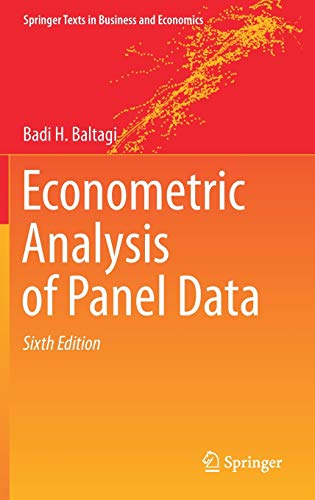# An R package with Baltagi’s ‘Econometric Analysis of Panel Data’ datasets

R
Linear models
Tidy datasets to let you focus on the econometric techniques rather than on data wrangling.
Author

Mauricio “Pachá” Vargas S.

Published

August 28, 2023‘Econometric Analysis of Panel Data’ (ISBN 978-3-030-53952-8) provides up-to-date coverage of basic panel data techniques, illustrated with real economic applications and datasets. However, importing the original data from the book into R is not straightforward because some of the datasets are available in Microsoft Word format. I provide the datasets in tidy format, expecting that this will allow students to focus on the econometric techniques rather than on data wrangling.

## Installation

You can install the development version of baltagi like so:

``remotes::install_github("pachadotdev/baltagi")``

## Example

Baltagi, Song and Jung (2001) investigated the productivity of public capital in each US state’s private output, which is discussed in section 9.6.1 of the book (Empirical Example: Nested States Public Capital Productivity).

The original dataset is in Microsoft Word format (DOCX). To read it, you would need to copy it in Notepad, save as CSV , and then import it into R to find that there are data wrangling steps involved to make it usable. Alternatively, you can read the DOCX file directly in R with the `readtext` package and then proceed to the data wrangling.

`baltagi` saves all those steps and you can jump directly to the econometric analysis.

``````library(baltagi)
library(plm)

fit <- plm(
log(gsp) ~ log(priv_cap) + log(hwy) + log(water) + log(util) + log(emp) +
unemp,
data = produc,
index = c("st_abb", "year")
)

summary(fit)``````
``````Oneway (individual) effect Within Model

Call:
plm(formula = log(gsp) ~ log(priv_cap) + log(hwy) + log(water) +
log(util) + log(emp) + unemp, data = produc, index = c("st_abb",
"year"))

Balanced Panel: n = 48, T = 17, N = 816

Residuals:
Min.    1st Qu.     Median    3rd Qu.       Max.
-0.1207980 -0.0228756 -0.0015757  0.0183999  0.1548035

Coefficients:
Estimate  Std. Error t-value  Pr(>|t|)
log(priv_cap)  0.23580111  0.02621777  8.9939 < 2.2e-16 ***
log(hwy)       0.07747252  0.03125904  2.4784   0.01341 *
log(water)     0.07821567  0.01500290  5.2134 2.391e-07 ***
log(util)     -0.11438336  0.01815255 -6.3012 4.992e-10 ***
log(emp)       0.79958666  0.02974239 26.8837 < 2.2e-16 ***
unemp         -0.00519584  0.00098018 -5.3009 1.510e-07 ***
---
Signif. codes:  0 '***' 0.001 '**' 0.01 '*' 0.05 '.' 0.1 ' ' 1

Total Sum of Squares:    18.941
Residual Sum of Squares: 1.0313
R-Squared:      0.94555